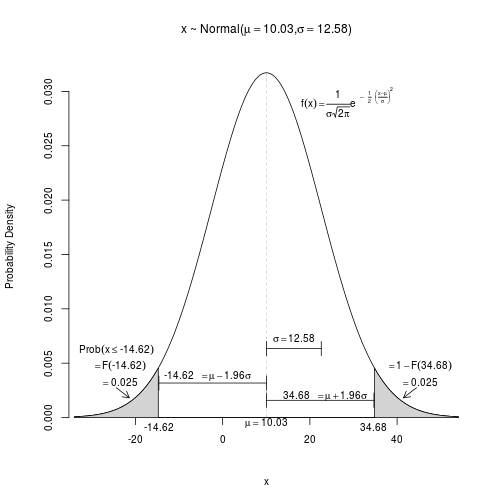### Filename: Normal1_2009_plotmathExample.R
### Paul Johnson June 3, 2009
### This code should be available somewhere in http://pj.freefaculty.org/R.  If it is not
### email me <pauljohn@ku.edu>

###Set mu and sigma at your pleasure:
mu <- 10.03
sigma <- 12.5786786

myx <- seq( mu - 3.5*sigma,  mu+ 3.5*sigma, length.out=500)

myDensity <- dnorm(myx,mean=mu,sd=sigma)

# It is challenging to combine plotmath with values of mu and sigma in one expression.
# Either bquote or substitute can be used.  First use bquote:

myTitle1 <- bquote (paste("x ~ Normal(", mu== .(round(mu,2)), ',', sigma== .(round(sigma,2)),")") )

### Using substitute:
### myTitle1 <-  substitute( "x ~ Normal" ~~ group( "(", list(mu==mu1, sigma^2==sigma2)#, ")") ,  list(mu1=round(mu,2), sigma2=round(sigma^2,2)))

### Or combine the two:
### t1 <- substitute ( mu == a ,  list (a = mu))
### t2 <- substitute ( sigma == a, list(a = round(sigma,2)))
### myTitle1 <- bquote(paste("x ~ Normal(", .(t1),",", .(t2),")" ) )

## To save the plot in a file, change FALSE to TRUE
SAVEME <- FALSE

if (SAVEME == TRUE){
pdf(file="Normal-2013.pdf", height=6.5, width=6.5, onefile=F, paper="special", pointsize=10)

}else {
##dev.new(height=6.5, width=6.5,pointsize=9)
}

## NULL

### xpd needed to allow writing outside strict box of graph
par(xpd=TRUE, ps=10)

plot(myx, myDensity, type="l", xlab="x", ylab="Probability Density ", main=myTitle1, axes=FALSE)
axis(2, pos= mu - 3.6*sigma)
axis(1, pos=0)
lines(c(myx,myx[length(myx)]),c(0,0)) ### closes off axes

# bquote creates an expression that text plotters can use
t1 <-  bquote( mu== .(mu))

# Find a value of myx that is "very close to" mu
centerX <- max(which (myx <= mu))
# plot light vertical line under peak of density
lines( c(mu, mu), c(0, myDensity[centerX]), lty= 14, lwd=.2)

# label the mean in the bottom margin
mtext(bquote( mu == .(mu)), 1, at=mu, line=-1)

### find position 20% "up" vertically, to use for arrow coordinate
ss = 0.2 * max(myDensity)
# Insert interval to represent width of one sigma
arrows( x0=mu, y0= ss, x1=mu+sigma, y1=ss, code=3, angle=90, length=0.1)

### Write the value of sigma above that interval
t2 <-  bquote( sigma== .(round(sigma,2)))
text( mu+0.5*sigma, 1.15*ss, t2)

### Create a formula for the Normal
normalFormula <- expression (f(x) == frac (1, sigma* sqrt(2*pi)) * e^{~~ - ~~ frac(1,2)~~ bgroup("(", frac(x-mu,sigma),")")^2})
# Draw the Normal formula
text ( mu + 0.5*sigma, max(myDensity)- 0.10 * max(myDensity),  normalFormula, pos=4)

### Theory says we should have 2.5% of the area to the left of: -1.96 * sigma.
### Find the X coordinate of that "critical value"
criticalValue <- mu -1.96 * sigma
### Then grab all myx values that are "to the left" of that critical value.
specialX <-  myx[myx <= criticalValue]

### mark the critical value in the graph
text ( criticalValue, 0 , label= paste(round(criticalValue,2)), pos=1)
### Take sequence parallel to values of myx inside critical region
specialY <- myDensity[myx < criticalValue]
#  Polygon makes a nice shaded illustration
polygon(c(specialX, specialX, specialX[length(specialX )]), c(0, specialY, 0), density=c(-110),col="lightgray" )

shadedArea <- round(pnorm(mu - 1.96 * sigma, mean=mu, sd=sigma),4)

### I want to insert annotation about area on left side.

al1 <- bquote(Prob(x <= .(round(criticalValue,2))))
al2 <- bquote(phantom(0) == F( .(round(criticalValue,2)) ))

### Hard to position this text "just right"
### Have tried many ideas, this may be least bad.
### Get center position in shaded area
medX <- median(specialX)
### density at that center point:
denAtMedX <- myDensity[indexMed <- max(which(specialX < medX))]

text(medX, denAtMedX+0.0055, labels=al1)
text(medX, denAtMedX+0.004, labels=al2)
text(medX, denAtMedX+0.0025, labels=al3)

### point from text toward shaded area
arrows( x0=medX, y0=myDensity[indexMed]+0.002 ,x1= mu-2.5 *sigma, y1= 0.40*myDensity[length(specialX)] ,   length=0.1)

ss <- 0.1 * max(myDensity)
### Mark interval from mu to mu-1.96*sigma
arrows( x0=mu, y0= ss, x1=mu-1.96*sigma, y1=ss, code=3, angle=90, length=0.1)
### Put text above interval
text( mu - 2.0*sigma,1.15*ss, bquote(paste(.(round(criticalValue,2)),phantom(1)==mu-1.96 * sigma,sep="")),pos=4 )

criticalValue <- mu +1.96 * sigma
### Then grab all myx values that are "to the left" of that critical value.
specialX <-  myx[myx >= criticalValue]

### mark the critical value in the graph
text ( criticalValue, 0 , label= paste(round(criticalValue,2)), pos=1)
### Take sequence parallel to values of myx inside critical region
specialY <- myDensity[myx > criticalValue]
#  Polygon makes a nice shaded illustration
polygon(c(specialX, specialX, specialX[length(specialX )]), c(0, specialY, 0), density=c(-110),col="lightgray" )

shadedArea <- round(pnorm(mu + 1.96 * sigma, mean=mu, sd=sigma, lower.tail=F),4)

### Insert simpler comment on right side.

al2 <- bquote(phantom(0) == 1 - F( .(round(criticalValue,2)) ))

### Hard to position this text "just right"
### Have tried many ideas, this may be least bad.
### Get center position in shaded area
medX <- median(specialX)
### density at that center point:
denAtMedX <- myDensity[indexMed <- max(which(specialX < medX))]

text(medX, denAtMedX+0.004, labels=al2)
text(medX, denAtMedX+0.0025, labels=al3)

### point from text toward shaded area
arrows( x0=medX, y0=myDensity[indexMed]+0.002 ,x1= mu+2.5 *sigma, y1= 0.40*myDensity[length(specialX)] ,   length=0.1)

ss <- 0.05 * max(myDensity)
### Mark interval from mu to mu+1.96*sigma
arrows( x0=mu, y0= ss, x1=mu+1.96*sigma, y1=ss, code=3, angle=90, length=0.1)
### Put text above interval
text( mu + 1.96*sigma,1.15*ss, bquote(paste(.(round(criticalValue,2)),phantom(1)==mu+1.96 * sigma,sep="")),pos=2 )##if (SAVEME == TRUE)  dev.off()